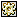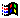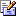Contents Articles Behaviors Books Director News Director Web Sites FAQ Games Mailing Lists News Groups Project Examples Reviews Software Tools Useful Web Sites Utilities Xtras

 Don't miss these#Export Global Variables

 Compatibilities:Rating:Author: MediaMacros (website)

Use this to export all of your global variables to a text file and then read them back in.

 --Copyright 1999 Chuck Neal --chuck@mediamacros.com --If you find this code helpful, send me an e-mail and let me know.  :-) on saveGlobalList theFileName   --set a variable delimiter   varDel = "New Variable Line Starts Here"   globalFile = ""   --create the content of the file   repeat with x = 1 to (the globals).count     globalFile = globalFile & varDel & return & "Value Type = " & (the globals)[x].ilk & return & "Value Name = " & string(getPropAt(the globals, x)) & return & (the globals)[x] & return     if x = (the globals).count then       globalFile = globalFile & varDel     end if   end repeat   --write the file   myFile = new(xtra "fileio")     if fileExists(theFilename) = 0 then     deleteFile(theFilename)   end if   createFile(myFile, theFilename)   openFile(myFile, theFilename, 2)     writeString(myFile, globalFile)     closeFile(myFile)                       myFile = 0   end on loadGlobals theFileName   --get the file contents   myFile = new(xtra "fileio")   if fileExists(theFilename) = 0 then     openFile(myFile, theFileName, 1)     fileContents = readFile(myFile)     closeFile(myFile)     myFile = 0   else     alert "File does not exist"   end if   valType = "Value Type = "   valName = "Value Name = "   theType = "string"   theName = ""   theValue = ""   varDel = "New Variable Line Starts Here"   repeat with x = 1 to fileContents.line.count     --check to see if you are starting a new variable and if so write the value to the variable     if fileContents.line[x] starts varDel and theName <> "" then       if theType = "string" then         (the globals)[symbol(theName)] = string(theValue)       else         (the globals)[symbol(theName)] = value(theValue)       end if       theType = "string"       theName = ""       theValue = ""     else  if fileContents.line[x] starts varDel and theName = "" then       --allows line 1 to write correctly       nothing     else       if fileContents.line[x] starts valType then         theType = (fileContents.line[x]).char[(valType.char.count + 1)..(fileContents.line[x].char.count)]       else if fileContents.line[x] starts valName then         theName = (fileContents.line[x]).char[(valName.char.count + 1)..(fileContents.line[x].char.count)]       else         if theValue = "" then           theValue = fileContents.line[x]         else           theValue = theValue & return & fileContents.line[x]         end if       end if     end if   end repeat end on getBehaviorDescription me   return = "This handler saves a test file with all global variables and can then re-import them.  This makes use of lingo"s -the globals- variable to get and set the global variables." end

 Contact MMI 36 South Court Sq Suite 300 Newnan, GA 30263 USASend e-mail